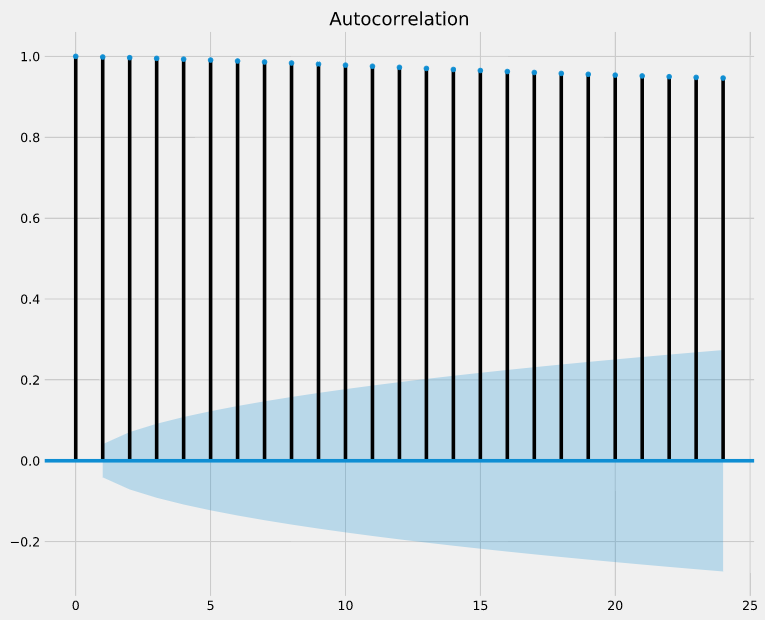# Interpret autocorrelation plots

If autocorrelation values are close to 0, then values between consecutive observations are not correlated with one another. Inversely, autocorrelations values close to 1 or -1 indicate that there exists strong positive or negative correlations between consecutive observations, respectively.

In order to help you asses how trustworthy these autocorrelation values are, the plot_acf() function also returns confidence intervals (represented as blue shaded regions). If an autocorrelation value goes beyond the confidence interval region, you can assume that the observed autocorrelation value is statistically significant.

In the autocorrelation plot below, are consecutive observations highly correlated (i.e superior to 0.5) and statistically significant?